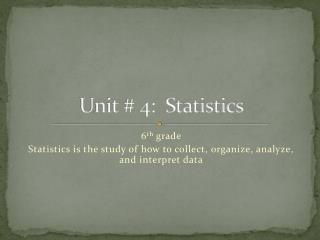Download PresentationUnit # 4: Statistics

# Unit # 4: Statistics - PowerPoint PPT PresentationDownload Presentation## Unit # 4: Statistics

- - - - - - - - - - - - - - - - - - - - - - - - - - - E N D - - - - - - - - - - - - - - - - - - - - - - - - - - -
##### Presentation Transcript

1. Unit # 4: Statistics 6th grade Statistics is the study of how to collect, organize, analyze, and interpret data

2. “We are rapidly entering a world where everything can be monitored and measured but the big problem is going to be the ability of humans to use, analyze, and make sense of the data.” • The New York Times • August 6, 2009

3. Essential Questions • 1. How do we properly collect data? • 2. How do we organize data? • 3. How do we analyze data? • 4. How do we interpret results?

4. To properly collect data, we must first ask a question that will result in multiple and varying answers rather than a single answer. A statistical question will result in variability in data and involve a real-world context.

5. Sort the following questions into two groups: statistical & non-statistical • 1. How old is the oldest student in our class? • 2. Are there more boys or girls in our class? • 3. How old are the students in our class? • 4. How long do students in this class spend on math homework each week? • 5. How many students in our class like to watch scary movies? • 6. What types of movies are preferred by students in our class?

6. Statistical or Non-Statistical Statistical • 3. How old are the students in our class? • 4. How long do students in this class spend on math homework each week? • 6. What types of movies are preferred by students in our class? Non-Statistical • 1. How old is the oldest student in our class? • 2. Are there more boys or girls in our class? • 5. How many students in our class like to watch scary movies?

7. Get into groups of 3 • Decide whether the questions in your envelope are statistical or non-statistical. Discuss. • If a question is non-statistical, discuss how you could rewrite it to be a statistical question that contains multiple and varying answers. • http://grade6commoncoremath.wikispaces.hcpss.org/Unit+5+Statistics+Probability • 6.SP.1 Questions for Sorting

8. Pick one of the following topics 1. TV habits 2. Cell phone usage 3. Eating fruits and vegetables 4. Watching sports Write a statistical question and a non-statistical question about your topic.